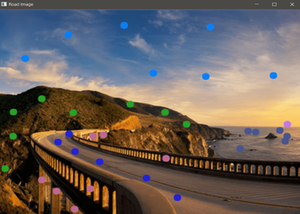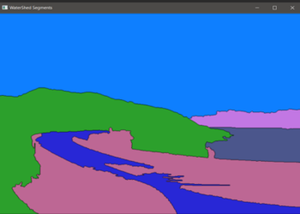# Contour Detection with Custom Seeds using Python – OpenCV

• Last Updated : 29 Oct, 2021

This article discusses how we can dynamically detect contours by clicking on the Image and assign different colors to different parts of the image. Contours are a very useful tool for shape analysis and object detection and recognition.  This program uses OpenCV, Numpy, and Matplotlib libraries.  It also uses a built-in OpenCV Watershed algorithm to detect contours.

### Requirements

• Python and OpenCV must be installed on the local machine.
• Install Jupyter Notebook for easy debugging.
• Here, Matplotlib’s colormap is used to get different colors. In the example given below, we will be using tab10. You can choose different colormaps. Refer to this site for different colors.

### Explanation

• Run the program.
• Click on the image where you want to make contours.
• Select a different color for a different part of the image by pressing numbers from zero to nine. (One number for one color)

Below is the Implementation.

## Python3

 `# Import modules``import` `cv2``import` `numpy as np``import` `matplotlib.pyplot as plt``from` `matplotlib ``import` `cm` `# Upload the image in the same directory and then run the code``# If image not found you may get an error``# Reading Image``road ``=` `cv2.imread(``'road_image.jpg'``)` `# Making Copy of Image``road_copy ``=` `np.copy(road)` `# Creating two black image of same size as original image``marker_image ``=` `np.zeros(road.shape[:``2``], dtype``=``np.int32)``segments ``=` `np.zeros(road.shape, dtype``=``np.uint8)` `# Function to return tuple of colors``def` `create_rgb(i):``    ``x ``=` `np.array(cm.tab10(i))[:``3``]``*``255``    ``return` `tuple``(x)`  `# Storing Colors``colors ``=` `[]` `# One color for each single digit``for` `i ``in` `range``(``10``):``    ``colors.append(create_rgb(i))` `# Global Variables``# Color Choices``# Number of markers``no_markers ``=` `10` `# Current markers``current_marker ``=` `1` `# Flag``marks_updated ``=` `False` `# CALLBACK FUNCTION``def` `mouse_callback(event, x, y, flags, param):``    ``global` `marks_updated` `    ``if` `event ``=``=` `cv2.EVENT_LBUTTONDOWN:``        ` `        ``# TRACKING FOR MARKERS``        ``cv2.circle(marker_image, (x, y), ``5``, (current_marker), ``-``1``)``        ` `        ``# DISPLAY ON USER IMAGE``        ``cv2.circle(road_copy, (x, y), ``5``, colors[current_marker], ``-``1``)` `        ``marks_updated ``=` `True`  `# Naming the window and setting call back function to it``cv2.namedWindow(``'Road Image'``)``cv2.setMouseCallback(``'Road Image'``, mouse_callback)` `while` `True``:``  ` `    ``# Show the 2 windows``    ``cv2.imshow(``'WaterShed Segments'``, segments)``    ``cv2.imshow(``'Road Image'``, road_copy)` `    ``# Close everything if Esc is pressed``    ``k ``=` `cv2.waitKey(``1``)``    ``if` `k ``=``=` `27``:``        ``break` `    ``# Clear all colors and start over if 'c' is pressed``    ``elif` `k ``=``=` `ord``(``'c'``):``        ``road_copy ``=` `road.copy()``        ``marker_image ``=` `np.zeros(road.shape[``0``:``2``], dtype``=``np.int32)``        ``segments ``=` `np.zeros(road.shape, dtype``=``np.uint8)` `    ``# If a number 0-9 is chosen index the color``    ``elif` `k > ``0` `and` `chr``(k).isdigit():``        ` `        ``# chr converts to printable digit``        ``current_marker ``=` `int``(``chr``(k))` `    ``# If we clicked somewhere, call the watershed``    ``# algorithm on our chosen markers``    ``if` `marks_updated:``        ``marker_image_copy ``=` `marker_image.copy()``        ``cv2.watershed(road, marker_image_copy)``        ``segments ``=` `np.zeros(road.shape, dtype``=``np.uint8)` `        ``for` `color_ind ``in` `range``(no_markers):``          ` `            ``# COLORING SEGMENTS``            ``segments[marker_image_copy ``=``=` `(color_ind)] ``=` `colors[color_ind]` `        ``marks_updated ``=` `False` `# Destroy all the windows at the end``cv2.destroyAllWindows()`

Output:

This code will open two windows. One with the original image and one black. Clicking on the original image will create small circles on it and the contours will show on the black image. (Press numbers from 0-9 to change colors and produce a different contour plot.)Original ImageProcessed Image

My Personal Notes arrow_drop_up